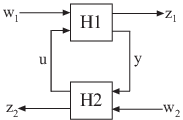Catalog of Model Interconnections

Each type of block diagram connection corresponds to a model interconnection command or arithmetic expression. The following tables summarize the block diagram connections with the corresponding interconnection command and arithmetic expression.

Model Interconnection Commands

Block Diagram ConnectionCommandArithmetic Expressionseries(H1,H2)H2*H1parallel(H1,H2)H1+H2parallel(H1,-H2)H1-H2feedback(H1,H2)H1/(1+H2*H1) (not recommended)N/AH1/H2 (division)N/AH1\H2 (left division)inv(H1)N/Alft(H1,H2,nu,ny) N/A

Arithmetic Operations

You can apply almost all arithmetic operations to dynamic system models, including those shown below.

Operation

Description

+

-

Subtraction

*

Multiplication

.*

Element-by-element multiplication

/

Right matrix divide

\

Left matrix divide

inv

Matrix inversion

'

Conjugate transposition. See ctranspose.

.'

Transposition

^

Powers of a dynamic system model, as in the following syntax for creating transfer functions:

s = tf('s');
G = 25/(s^2 + 10*s + 25);

In some cases, you might obtain better results using model interconnection commands, such as feedback or connect, instead of model arithmetic. For example, the command T = feedback(H1,H2) returns better results than the algebraic expression T = H1/(1+H2*H1). The latter expression duplicates the poles of H1, which inflates the model order and might lead to computational inaccuracy.